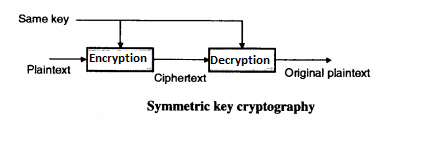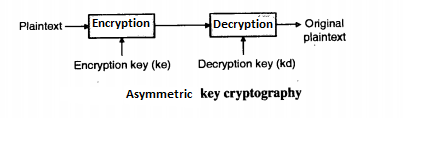# Types Of Cryptography – Symmetric And Asymmetric Key Cryptography (All You Want To Know !!)

Security is an important aspect of the networking world . Security in networking is based on the means of  Cryptography . The word cryptography comes from the Greek word (which means hidden or secret). Cryptography is the art as well as science of secret writing of information/message and makes them non-readable.

The process of transformation/conversion of plaintext message into ciphertext message is called encryption and the process of transformation/conversion of ciphertext message into plaintext message is called Decryption .

Cryptography can provide confidentiality, integrity, authentication, and nonrepudiation of messages. Cryptography  is mainly based upon the principles of abstract algebra (though in this post we tried to keep it theoretical to a large extent) .

Simply in any cryptography process, the initial plaintext will be encrypted to a ciphertext and ultimately on the receiver side, with the decryption process the ciphertext again gets converted into plaintext .

The original message, before being transformed, is called plaintext. After the message is transformed, it is called ciphertext. An encryption algorithm transforms the plaintext into ciphertext, a decryption algorithm transforms the ciphertext back into plaintext. The sender uses an encryption algorithm, and the receiver uses a decryption
algorithm .

We can divide all the cryptography algorithms (ciphers) into two groups: symmetric key (also called a secret key) cryptography algorithms and asymmetric (also called public key) cryptography algorithms .## What Is Symmetric Key Cryptography ?

Symmetric key cryptography is also called as secret key cryptography or private key cryptography. In secret-key cryptography, a single key is used for encryption as well as decryption. Encryption and decryption process uses the same key .It is represented as P = D (K, E(P)).
Where P = Plain Text, E (P) = Encryption of plain text, D = Decryption, K = key

For Example : Stream and block cipher. Data Encryption Standard (DES), Advance Encryption Standard (AES) and BLOFISH.

As the same key is used for encryption and decryption receiver must have the sender’s key , without which the receiver cannot decrypt it (without sender’s permission).

Symmetric key achieves the authentication principle because it checks receivers’ identity. DES and AES techniques are implemented using symmetric key cryptography. System resources are less utilized in symmetric key cryptography .

#### What Are Some Advantages & Disadvantages Of Symmetric Key Cryptography ?

Below are some of the merits of Symmetric Cryptography :

The symmetric key is faster than asymmetric key cryptography. Because single key data cannot decrypt easily at the receiver side even if it is intercepted by attacker.

As the same key is used for encryption and the decryption receiver must have the senders key he cannot decrypt it without sender permission. The symmetric key achieves the authentication principle because it checks receivers’ identity.

Now some demerits of Symmetric Cryptography :

Once the key is stolen while transmitting data between the sender and receiver it is very easy to decrypt the message as the same key is used for encryption and decryption.

In symmetric key cryptography, the key is transmitted first and then the message is transferred to the receiver. If the attacker intercepts the communication between the sender and receiver then he/she can decrypt the message before it reaches intended recipients.

#### What Is Asymmetric Key Cryptography?

Asymmetric key cryptography is also called as public key cryptography or conventional cryptographic system. In asymmetric key cryptography, two keys are used, one is for encryption, and the other is for decryption.It is represented as P = D(Kd, E (Ke,P))
Where P = Plain Text, E (P) = Encryption of plain text, D = Decryption, Ke and Kd = Encryption key and Decryption Key.

For Example : Rivest Shamir Adlman (RSA) and Diffie Hellman key exchange algorithm

In public-key encryption/decryption, the public key that is used for encryption is different from the private key that is used for decryption. The public key is available to the public the private key is available only to an individual receiver .

Note : In symmetric-key cryptography, the same key locks and unlocks the box (message). In asymmetric-key cryptography, one key locks the box, but another key is needed to unlock it.

#### What Are Some Advantages & Disadvantages Of Asymmetric Key Cryptography ?

Some merits of Asymmetric key cryptography can be seen as follows :

In the Asymmetric key cryptography key cannot be distributed among sender and receiver as both have their own key so there is no problem of key distribution while transmitting the data over an insecure channel.

The main advantage of asymmetric key cryptography is that two separate keys are used for used encryption and decryption; even if the encryption key is stolen by attacker he/ she cannot decrypt the message as the decryption key is only available with receiver only.

Now some demerits of this type of cryptography are as follows :

Different keys used between the sender and receiver requires more time to get the transmission done as compared to symmetric key cryptography. (Slower than symmetric key cryptography very few asymmetric encryption methods achieve the fast transmission of data).

Asymmetric key cryptography utilizes more resource as compare to symmetric key cryptography.

Note : Please note that there are total three types of Keys as mentioned above used in Cryptography i.e. Secret key (used in Symmetric Cryptography) and also we have public and private key as used in the Asymmetric cryptography

Hope you really liked this post about the Symmetric and Asymmetric types of encryption in cryptography. Stay tuned for more interesting stuff in this series .